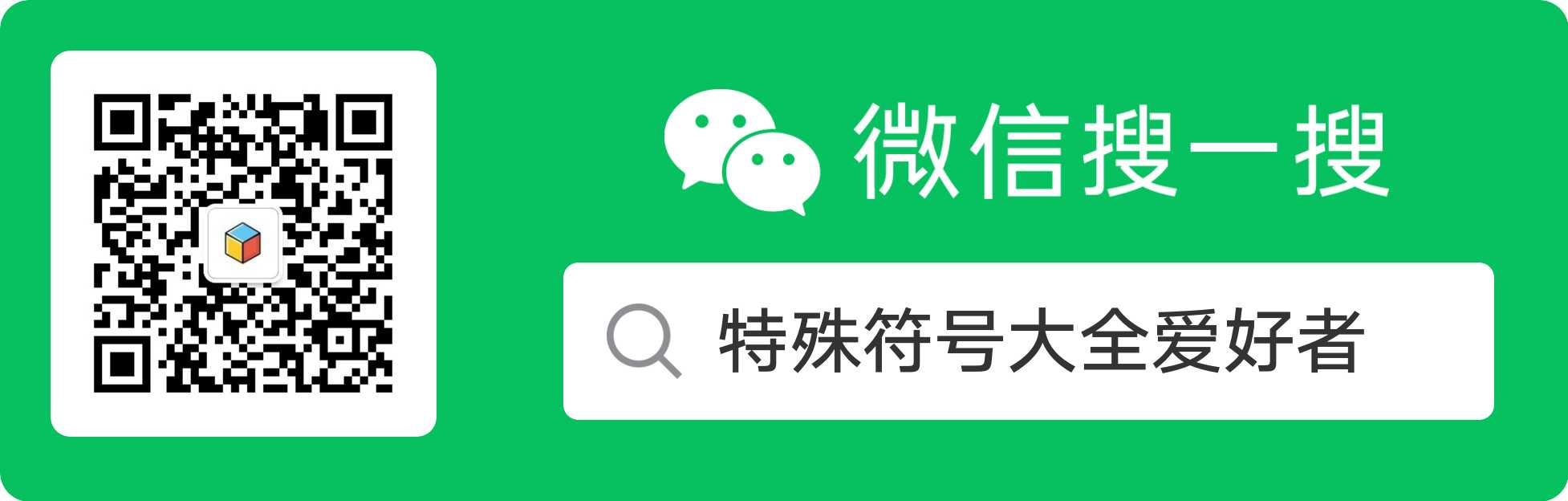# 生气颜文字表情符号

▄︻┻┳═一…… ☆（>○<）

o(≧口≦)o

(⊙x⊙;)

￣へ￣

（＃￣～￣＃）

(*￣︿￣)

(* ￣︿￣)

ヽ（≧□≦）ノ

<)。(>

___*( ￣皿￣)/#____

(￣ε(#￣)☆╰╮o(￣皿￣///)

<( ￣^￣)(θ(θ☆( >_<

→)╥﹏╥)

(ﾟДﾟ*)ﾉ

○|￣|_ =3

┻━┻︵╰(‵□′)╯︵┻━┻

(；′⌒`)

o(￣ヘ￣o＃)

(o ￣3)==@))゜ロ゜((@==(′ε′ )o

<(￣ ﹌ ￣)@m 就你！

(〃＞目＜)

（＝。＝）

(///￣皿￣)○～

<( ‵□′)>───Ｃε(┬﹏┬)3

ε=怒ε=怒ε=怒ε=怒ε=( o｀ω′)ノ

▄︻┻┳═一……

~~( ﹁ ﹁ ) ~~~

φ(-ω-*)

(ーー゛)

(╬￣皿￣)＝○＃(￣＃)３￣)

(-__-)=@))> o<)

(。・・)ノ

▄︻┻┳═一∵∴∷∶∵ (∵_,∵)>>>>

（｀へ´）

(ー`´ー)

<(－︿－)>

( ｀д′)

z(-_-z)).....((s-_-)s